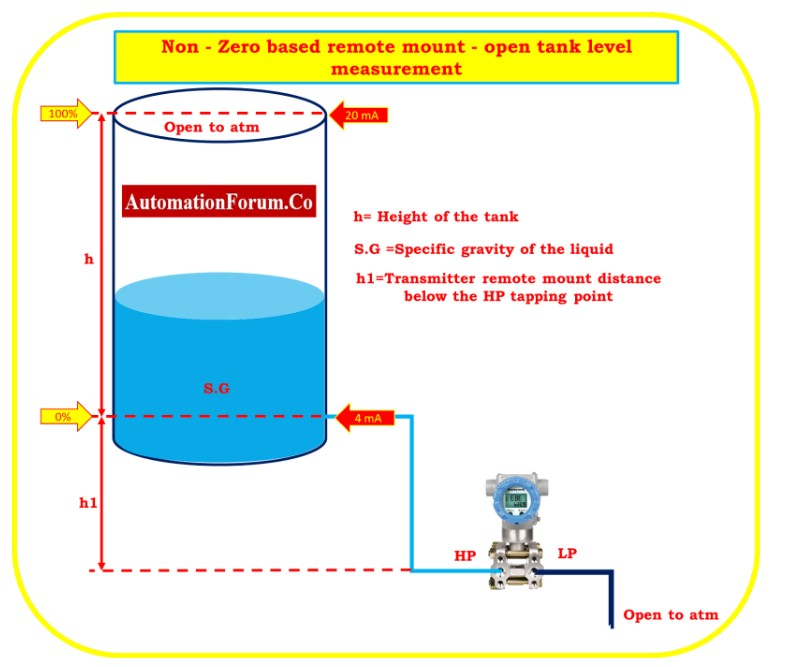# DP calculator for Zero suppression – open tank level measurementThere are two types of mounting possible in the suppression type level measurement as shown below

## Non – Zero based direct mount – open tank level measurement

The level transmitter is positioned in the same level position as the HP tapping point when it is connected. But the minimum range of the level is above the HP tapping point.

## Non – Zero based remote mount – open tank level measurement

In the tank, the level transmitter was installed in a location that was below the HP tapping point.

## LRV Calculation

When the tank is filled with minimum level h1(0%),

DP = Patm+(SG*h)+(SG*h1) – Patm

PH.P = Patm+(SG*h)+(SG*h1)

When level is at zero h=0

= Patm+(SG*0)+(SG*h1)

PH.P =Patm+(SG*h1)

PL.P = Patm

LRV = PH.P. –  PL.P

= Patm+(SG*h1) – Patm

LRV=(SG*h1)

## Estimating the URV

When the tank is completely full of liquid (h), determine the transmitter’s differential pressure (DP).

PH.P  = Patm +(SG*h)+(SG*h1)

PL.P  = Patm

URV  =  P.HP –  P.LP

= Patm+(S.G*h)+(SG*h1) – Patm

URV = (S.G*h)+(SG*h1)

Range of the transmitter  = (SG*h1) to (S.G*h)+(SG*h1)